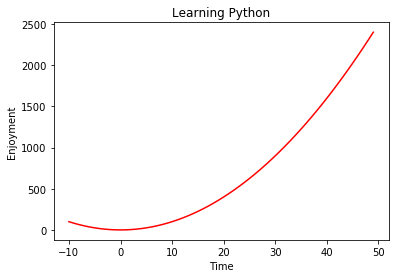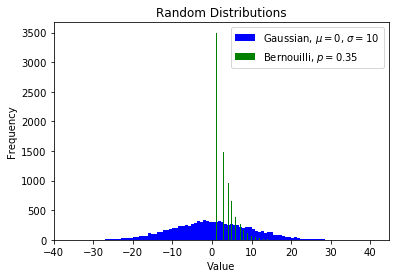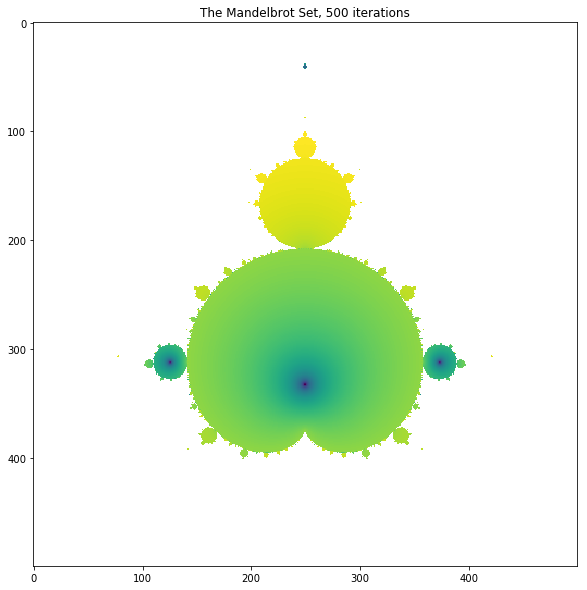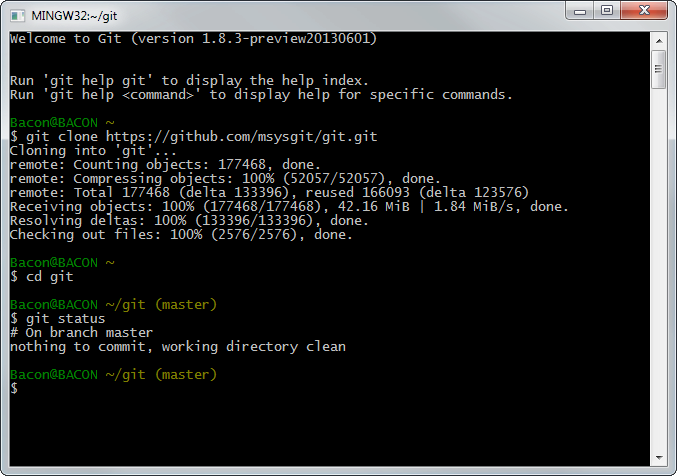# Introduction to the Python Scientific Ecosystem, Part 2

Posted on August 10, 2017 in PythonWith the rise in popularity of Python as a tool for data analysis, I'd like to spend some time showing you some tools that have recently emerged as new 'standards' for computation in science and engineering.

This post is the second in a series. You might wish to take a look at the first installment before reading this one.

The second part will talk about 'what' Python is used for -- that is, a primer on the standard scientific 'stack' of Python packages and libraries that are now part of a open source stack used in many companies, universities and departments.

## Jupyter Notebooks¶Building on IPython, Jupyter Notebooks are an in-browser way to structure code and text in a way that is easy to read and follow -- in fact, you're reading one right now! Even if the reader doesn't "get" 100% of the code, it's still possible to understand the train of thought and how the analyst got to his or her conclusions. This style of programming is also commonly referred to as "literate programming" (by Donald Knuth). She can then run the code herself, changing things here and there to better understand what's going on. Finally, she may be able to take that code and add to it, or reuse it elsewhere in her work.

From WIkipedia:

Literate programs (LPs) are written as an uninterrupted exposition of logic in an ordinary human language, much like the text of an essay, in which macros are included to hide abstractions and traditional source code.

LP tools are used to obtain two representations from a literate source file: one suitable for further compilation or execution by a computer, the "tangled" code, and another for viewing as formatted documentation, which is said to be "woven" from the literate source.

A Notebook is structured in cells, that is, little units of either text or code, like this:

In :
print('Hello World!')

Hello World!

In :
import math
print(math.pi)

3.141592653589793

In :
# Lists are made with square brackets
numbers = [0, 1, 2, 3, 4, 5, 6, 7, 8, 9]
print(numbers)

# They can contain basically anything
kitchen_sink = [math.e, 'words', numbers, -4]
print(kitchen_sink)

[0, 1, 2, 3, 4, 5, 6, 7, 8, 9]
[2.718281828459045, 'words', [0, 1, 2, 3, 4, 5, 6, 7, 8, 9], -4]

In :
# You can access list members by 'slicing' with square brackets.
# The syntax is [starting index: ending index(: stride)]
print(numbers)

# Slice multiple members - note Python is zero-indexed
print(numbers[2: 4])

# Get every second element
print(numbers[::2])

# Negative numbers refer to indices starting from the end
print(kitchen_sink[-2])

3
[2, 3]
[0, 2, 4, 6, 8]
[0, 1, 2, 3, 4, 5, 6, 7, 8, 9]


Python has other data structures. You will need to take the time to understand how they work when you get there.

In :
# Dictionaries are used to store 'database-like' information
satellites_status = {'R1': 'Dead', 'R2': 'Operational'}
print('R2 Status: ', satellites_status['R2'])

# Tuples are immutable containers of fixed size, great for passing data around
rgb_fuchsia = (255, 0, 255)
print('RGB Values for fuchsia:', rgb_fuchsia)

# Sets are unique collections of items, and set-theoretic
# operations (AND, OR, etc.) can be applied to them.
group = [1, 4, 6, 8, 9, 3, 4, 6, 1, 3, 5, 1, 2, 6, 1, 0]
print(set(group))

R2 Status:  Operational
RGB Values for fuchsia: (255, 0, 255)
{0, 1, 2, 3, 4, 5, 6, 8, 9}

In :
# There's also many shorthand ways to things with Python
for n in range(10):
print(n)

0
1
2
3
4
5
6
7
8
9

In :
# You can define functions. These transform items passed to them
def square(n):
"""Given number n, returns square of that number."""
return n ** 2

for n in range(10):
print(n, 'squared is', square(n))

0 squared is 0
1 squared is 1
2 squared is 4
3 squared is 9
4 squared is 16
5 squared is 25
6 squared is 36
7 squared is 49
8 squared is 64
9 squared is 81

In :
# But make sure you're calling the right item types to your functions
# Python doesn't know how to square a list, for example.
print(square(range(10)))

---------------------------------------------------------------------------
TypeError                                 Traceback (most recent call last)
<ipython-input-8-932aa55faf8a> in <module>()
1 # But make sure you're calling the right item types to your functions
2 # Python doesn't know how to square a list, for example.
----> 3 print(square(range(10)))

<ipython-input-7-d5c443d88be8> in square(n)
2 def square(n):
3     """Given number n, returns square of that number."""
----> 4     return n ** 2
5
6 for n in range(10):

TypeError: unsupported operand type(s) for ** or pow(): 'range' and 'int'

You can also make use of IPython's special abilities, for example by calling the pwd UNIX command, print working directory, by preceding it with an exclamation mark:

In :
!pwd

/c/Users/LeblancFR/notebooks/blog


Jupyter provides an easy way to plot images alongside the text, too. There's a whole section on that later.

In :
import matplotlib.pylab as plt

# Create a list of numbers -10 to 49
x = range(-10, 50)
# Create a new list that takes every member of x and squares it
y = [square(n) for n in x]

# Plotting stuff, which we get into next section.
plt.figure()
plt.plot(x, y, 'r')
plt.xlabel('Time')
plt.ylabel('Enjoyment')
plt.title('Learning Python')
plt.show()Text is rendered from Markdown (see chapter below), and code can be either Julia, Python or R (hence the name, ju-py-r). To interact with the notebooks, the program "Jupyter Notebook Server" needs to be running on your computer, as a way for the Python interpreter to interact with the code inside your browser. This way, it eliminates the need to run Python code from the command line and text editor.

It is to be used for exploration, exposition, visualization, noodling, etc., but it is not useful to write "production" code. In fact, many scientific and engineering books are now written in Jupyter Notebooks, and later ported to PDF and paper. You can try yourself online through Jupyter's website. Hundreds of interesting notebooks can also be found here. For me, it all started with this one:

A note on history, Jupyter notebooks was originally called 'IPython notebooks', and a lot of good documentation and books has been written refering to it in that way. It is useful to know when googling for answers.

### Markdown¶Markdown is a human-readable way to annotate plain text so that it can be parsed to produce HTML or HTML-like code. The syntax is extremely easy to pick up, and provides a powerful way of expressing rich text with little "overhead". In fact, if you've ever written or edited text on Wikipedia (and tons of other sites), you've probably already been using it without knowing. It is very powerful. Markdown text, and in fact whole Jupyter Notebooks, can be transformed to and from multiple sources, including PDF, HTML, DOC, PPT, and many, many others.

The original specification can be found on the original website, and further details specific to Jupyter can be found here.

In a nutshell:

• Italics is one asterisk: *italics* -> italics
• Bold is two asterisks: **bold** -> bold
• Lines with preceding with "# " will be formatted as headers. e.g. this cell's header is: ### Markdown
• Code blocks inline with text can be enclosed in backticks. words gives words
Code sections (whole lines) can either be surrounded by \\\ or ~~~, or preceded by four spaces or more.
• Special characters ($\*_{}[]()#+-.!) can escaped with a backslash, e.g. \$ 400 gives \$400 Quoted text lines can be preceded by the character >. • Links by placing alternating square brackets and parentheses: [click here](http://example.org) -> click here • Images by placing an exclamation point before a link to an image file: ![RADARSAT-1](https://upload.wikimedia.org/wikipedia/commons/thumb/b/bb/Radarsat-1.gif/260px-Radarsat-1.gif) -> ![RADARSAT-1](https://upload.wikimedia.org/wikipedia/commons/thumb/b/bb/Radarsat-1.gif/260px-Radarsat-1.gif) Jupyter Markdown cells can also use$\LaTeX$formatting for text, labels and equations by enclosing it in dollar signs: $\sqrt{evil}$ gives$\sqrt{evil}$. It can also create inline tables with dead-simple syntax: | Movie Title | Budget | Year | |--------------------------------|---------------|--------| | Something Completely Different | £80 000 | 1971 | | Holy Grail | \$400 000    |  1975  |
|  Life of Brian                 |  \$4 million | 1979 | | The Meaning of Life | \$9 million  |  1983  |


Gives:

Movie Title Budget Year
Something Completely Different £80 000 1971
Holy Grail \$400 000 1975 Life of Brian \$4 million 1979
The Meaning of Life \$9 million 1983 For better manipulatability, images can also be placed inline either with a traditional <img src="filename.jpg"> method with width, height, etc. attributes. In fact, most inline HTML tag, such as <br>, <div>, etc. will be directly interpreted by Markdown. ### Matplotlib¶Matplotlib is a 2D plotting library that is relatively easy to use, to create publication-quality plots. It provides an interface that is easy to get started with as a beginner, but it also allows you to customize almost every part of a plot. • Easy to get started • Support for$\LaTeX$formatted labels and texts • Great control of every element in a figure, including figure size and DPI. • High-quality output in many formats, including PNG, PDF, SVG, EPS, and PGF. • GUI for interactively exploring figures and support for headless generation of figure files (useful for batch jobs). • Supports add-on toolkits for specialty requirements (e.g. Cartopy for geospatial plotting, 3D plotting with mplot3d, etc.) To be perfectly honest, the package takes some getting used to. It is the de-facto plotting standard in Python however, and the time investment to learn it is rapidly worth it. It has bindings integrated with Jupyter Notebook, as well as many other libraries out there. Further reading: In : # Package imports.-------- import numpy as np # We'll talk about this one in the next chapter # We can interact with matplotlib.pylab objects directly with shorthand plt. # This simply saves typing, and is standard practice for often-used libraries. import matplotlib.pylab as plt # Create dummy data.-------- # Two different random distributions of 10'000 numbers. # In Jupyter, you can learn more about the functions' documentation, and # what arguments they use by Shift-Tabbing anywhere inside the parentheses. x = np.random.normal(loc=0.0, scale=10.0, size=10000) y = np.random.geometric(p=0.35, size=10000) ## Matplotlib starts here.-------- # We create a new pylab figure object, which we'll define in the following lines plt.figure() # Create 2 histograms from arrays x and y with the following attributes. # Notice the LaTeX in the label, denoted by surrounding $ symbols.
plt.hist(x, bins=100, color='blue', label=r'Gaussian, $\mu=0$, $\sigma=10$')
plt.hist(y, bins=100, color='green', label='Bernouilli, $p=0.35$')

# Next, we specify some figure attributes. These are quite self-explanatory.
plt.xlabel('Value')
plt.ylabel('Frequency')
plt.title('Random Distributions')
plt.legend()

# Finally, we "show" the whole thing. In Jupyter this outputs under the cell.
# Calling this in a script, instead, would open a new GUI window.
# All this in 10 lines of code!
plt.show()## Numpy¶NumPy is the de-facto standard for manipulating array-type data in Python, especially used for scientific purposes. It is built on top of C and FORTRAN, and offers simple Python bindings to work with data with vectorized operations. It gets its speed and memory efficiency by allowing series and arrays to contain data that is of one type only, be that integer, float, etc. Comparatively, a Python list might contain a mix of strings, ints, lists, etc. and must make a type check every time it needs to perform an action.

To understand Numpy, you first need to get acquainted with its basic unit, the ndarray. An ndarray is

• a regular grid of N-dimensions,
• homogeneous by default (all the elements have the same type),
• contiguous block of memory with types corresponding to machine types (8-bit ints, 32 bit floats, 64-bit longs, ...).

Numpy is the standard building block of almost all other packages mentioned in this series.

In :
import numpy as np

numbers = np.array([0, 1, 2, 3, 4, 5, 6, 7, 8, 9])
print(numbers)

[0 1 2 3 4 5 6 7 8 9]


#### Inspecting array properties¶

In :
# Numpy arrays come with an impressive list of "dot-methods"
# that can be called directly from the objects
print('Mean:        ', numbers.mean())

# You can discover these in Jupyter by pressing Tab with the
# cursor after the dot, say in numbers.|
print('ndarray type:', numbers.dtype)
print('Dimensions:  ', numbers.ndim)
print('Shape:       ', numbers.shape)

Mean:         4.5
ndarray type: int32
Dimensions:   1
Shape:        (10,)

In :
# There are also shorthand ways of accomplishing basic tasks
# such as creating a list of numbers from a range
numbers = np.arange(10)
print(numbers)

[0 1 2 3 4 5 6 7 8 9]

In :
# You can nest arrays, so that they create matrices
data = np.array([[1, 2, 3, 4, 5],
[1, 2, 3, 4, 5],
[1, 2, 3, 4, 5],
[1, 2, 3, 4, 5],
[1, 2, 3, 4, 5]])
print('Columns means:', data.mean(axis=0))
print('Row means:    ', data.mean(axis=1))

Columns means: [ 1.  2.  3.  4.  5.]
Row means:     [ 3.  3.  3.  3.  3.]

In :
# You can pass arrays through functions, and it will
# respect its type, and can include rounding and overflow
def square(n):
"""Given number n, returns its square."""
return n ** 2

print(square(numbers))

[ 0  1  4  9 16 25 36 49 64 81]


#### Slicing¶

We can use Python's [] operator to slice and dice the array. Slices share memory with the original array, so any operations made on them also affects the original array.

In :
print(data[0,0])  # First row, first column
print(data)    # The whole second row
print(data[:,2])  # The third column
print('---')
data[0, 0] = 9999 # Change value of first row, first col
print(data)

1
[1 2 3 4 5]
[3 3 3 3 3]
---
[[9999    2    3    4    5]
[   1    2    3    4    5]
[   1    2    3    4    5]
[   1    2    3    4    5]
[   1    2    3    4    5]]


#### Numpy for scientific computing - an example¶

Let's see how numpy can simplify our thought process when handling complex data. As an example, we'll create the Mandelbrot Set figure, starting only from its mathematical equation.

From Wikipedia:

The Mandelbrot set is the set of complex numbers $c$ for which the function $f_c(z)=z^2+c$ does not diverge

when iterated from $z=0$, i.e., for which the sequence $f_c(0)$, $f_c(f_c(0))$, etc., remains bounded in absolute value.

We can use numpy to create a 4-line program to calculate the Mandelbrot Set, which we'll then plot. Since by definition, Mandelbrot's Set uses the concept of recursion, we'll be using a pair slightly fancier Python functions called reduce and lambda. Don't get too caught up in what those functions precisely do right away - for the sake of the example, the goal is simply to understand that the function z is "calling itself" 20 times.

From Python's documentation on reduce():

For example, reduce(lambda x, y: x+y, [1, 2, 3, 4, 5], 0) calculates (((((0+1)+2)+3)+4)+5).

In :
from functools import reduce
import numpy as np
import matplotlib.pyplot as plt
from tqdm import tqdm

# Some pixels will overload (diverge), but that's the point
# So we'll hide the warning
import warnings
warnings.filterwarnings('ignore')

# Increase this to improve the shape of the fractal
iterations = 500

x, y = np.ogrid[-2:1:500j, -1.5:1.5:500j]
c = x + 1j * y

z = reduce(lambda x, y: x*x + c,  * iterations, c)

plt.figure(figsize=(10, 10))
plt.title('The Mandelbrot Set, {} iterations'.format(iterations))
plt.imshow(np.log(np.abs(z)))
plt.show()## Exporting the source code¶

Once you've finished doodling in notebooks, quite often the next step will be to move the contents of the code into a script that can be called as and when required -- either manually by running it in Windows Explorer, calling it from the Terminal (CMD.exe), or even by scheduling the script to be run on a periodical basis through Windows Task Scheduler.

You can export the contents of your notebook into a .py file easily. It will also bundle the contents of the Markdown cells into Python comments.

Maybe you'd like your code to now run as a script on your computer, or someone else's. Maybe you'd like Windows Scheduler to run them at a certain frequency, or maybe you'll be running them every time you do a certain task. To do this, you will usually want to tweak the code so that it performs better as a stand-alone Python scripts. Best practices for turning Jupyter notebooks into Python scripts can be found here.

### Version control - Git¶Once you've generated source code, it will need to be maintained for the future. Ways with which to do this fill up entire books, but it pays in the long run to spend some time storing you and your team's code properly. The first step towards this is to use a version control system. At the base, however, they all run on a program called git, that takes care to let multiple team members work on the same codebase simultaneously, without creating conflicts.

From Wikipedia:

Git is a version control system for tracking changes in computer files and coordinating work on those files among multiple people. It is primarily used for source code management in software development, but it can be used to keep track of changes in any set of files. As a distributed revision control system it is aimed at speed, data integrity, and support for distributed, non-linear workflows.

Modern versions of this will include bug trackers, test runners, integrated wikis, etc. The most well-known of these is the website https://github.com, which has single-handedly catalyzed a second-coming of open source software in the past decade.

On Windows machines, I would highly recommend you take a look at the git-for-Windows project, which installs a user-local version of the Cygwin bash environment, and provides the Windows user with a fully-functional unix shell, with grep, vim, curl, find, etc., and of course, git.